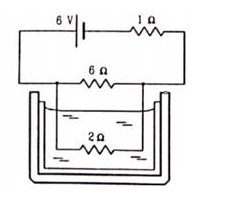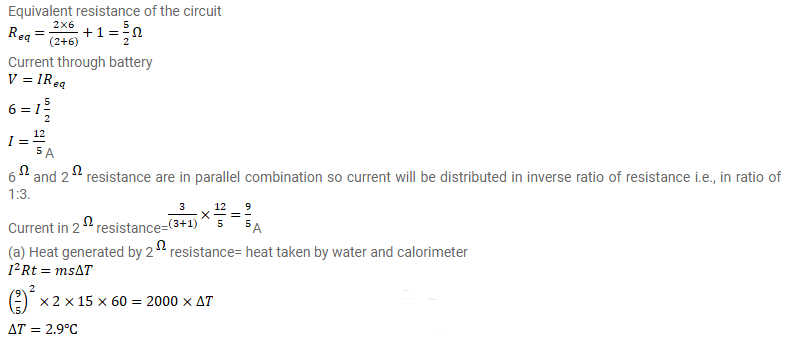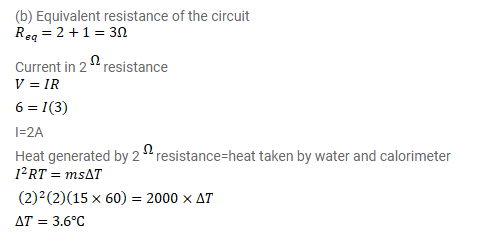# The 2.0 Ω resistor shown in figure is dipped into a calorimeter containing water.

Question:

The $2.0 \Omega$ resistor shown in figure is dipped into a calorimeter containing water. The heat capacity of the calorimeter together with water is $2000 \mathrm{~J} / \mathrm{K}$. (a) If the circuit is active for 15 minutes, what would be the rise in the temperature of the water? (b) Suppose the $6.0 \Omega$ resistor gets burnt. What would be the rise in the temperature of the water in the next 15 minutes?Solution: